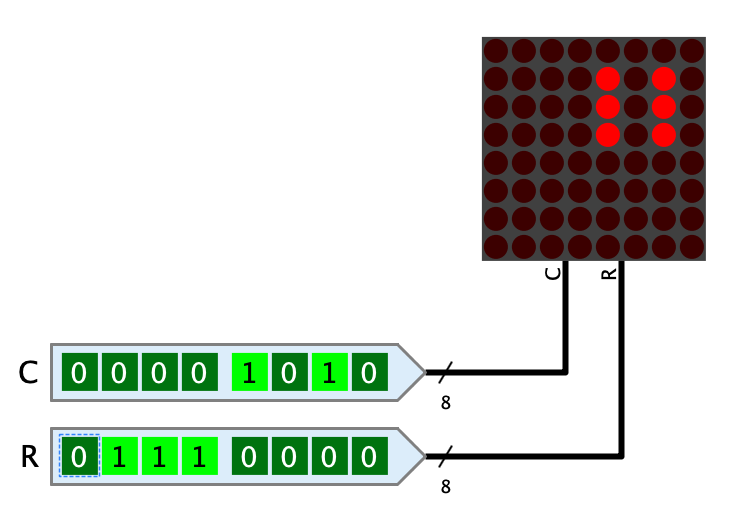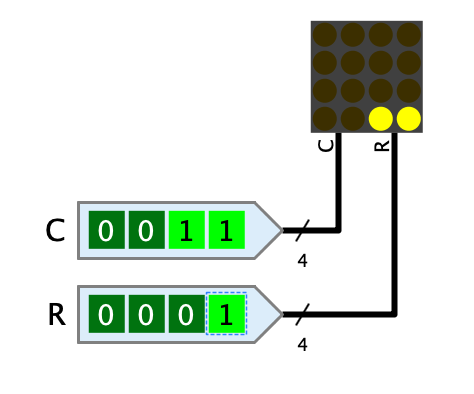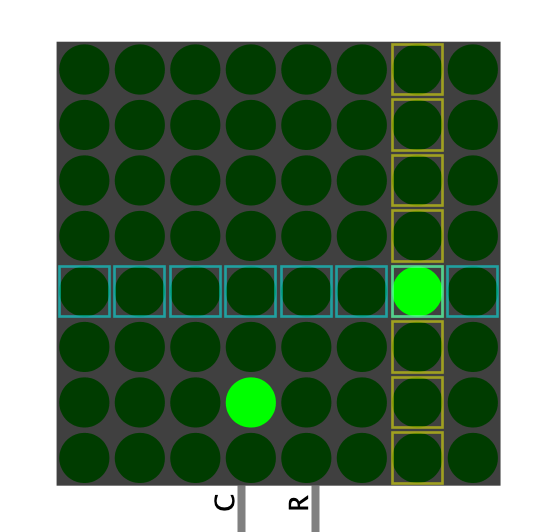## Appearance## Behavior

Represents a matrix of LEDs that may or may not be lit depending on the signals at the inputs. The matrix can have up to 32 rows and columns.

In order to address all light dots of a 32x32 matrix individually, the LED matrix would have to have an extremely large number of pins. For this reason, an addressing mode is used in which only the rows and columns are addressed. At the crossing points where both signals contain a 1, the light dot is lit.

Example: For a 4x4 LED matrix whose C-pin has the value 0011 and whose R-pin has the value 0001, the two left-hand dots in the bottom row are lit.This example shows that with this addressing mode, of course, not all dots can be addressed individually without further ado. In order to make this possible, the LED matrix is operated together with a multiplexing circuit that makes use of the afterglow time of LEDs. Antares' standard library contains the circuit "8x8 LED matrix with Ctrl" in the "Output" directory, which shows how such a multiplexing circuit works.

## Pins

C

The value that addresses the columns of the LED matrix. The least significant bit addresses the leftmost column. The bit width of the pin is defined by the value of the attribute "number of columns".

R

The value that addresses the rows of the LED matrix. The least significant bit addresses the bottom row. The bit width of the pin is defined by the value of the attribute "number of rows".

## Attributes

LED Color

The color of the LEDs. The menu offers all light colors implemented in Antares for selection.

Size

The LED matrix can be displayed in the three sizes "large", "medium" and "small".

Number of columns

Determines the number of columns of the LED matrix. The attribute can have a value between 1 and 32, which also determines the bit width of the "C" pin.

Number of rows

Determines the number of rows of the LED matrix. The attribute can have a value between 1 and 32. This value also determines the bit width of the "R" pin.

Afterglow duration (ms)

The duration in milliseconds that a light dot continues to glow after the corresponding values at the inputs have been set to 0. If the LED matrix is operated in multiplexing mode, this value must be matched to the frequency at which the input values change.

Draw dot as circle

Normally Antares draws the light dots as small rectangles without any space between the individual dots. If this attribute is set, small circles are drawn instead.

Debug

If this attribute is set, the LED matrix shows during simulation which row and columns at the inputs have the value 1 at the moment, which can be helpful for debugging, but also for educational purposes, if e.g. a multiplexing circuit is developed.